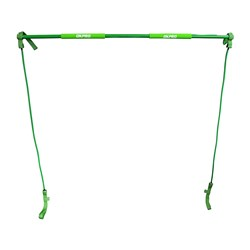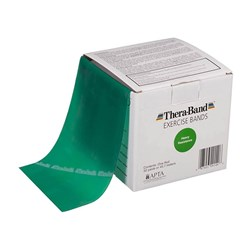# Resistance Training

Grid List
\$7.15
Increase value Decrease value
\$19.99
Increase value Decrease value
\$19.99
Increase value Decrease value
\$12.99
Increase value Decrease value
\$17.00
Increase value Decrease value
\$19.99
Increase value Decrease value
\$34.99
Increase value Decrease value
\$19.99
Increase value Decrease value
\$99.99
Increase value Decrease value
\$39.99
Increase value Decrease value
Practitioner Tubing 30m [Silver-Super-Strong]
\$115.60
Increase value Decrease value
\$169.99
Increase value Decrease value
\$499.00
Increase value Decrease value\$1507.00
Increase value Decrease value
\$8.80
Increase value Decrease value\$16.50
Increase value Decrease value
\$66.50
Increase value Decrease value
\$19.90
Increase value Decrease value\$198.00
Increase value Decrease value
\$33.69
Increase value Decrease value
\$23.71
Increase value Decrease value
\$13.83
Increase value Decrease value
\$4.60
Increase value Decrease value
From
Increase value Decrease value
From
Increase value Decrease value
From
Increase value Decrease value
From
Increase value Decrease value
From
Increase value Decrease value
From
Increase value Decrease value
From
Increase value Decrease value
From
Increase value Decrease value
From
Increase value Decrease value
From
Increase value Decrease value
From
Increase value Decrease value
From
Increase value Decrease value
From
Increase value Decrease value
From
Increase value Decrease value
From
Increase value Decrease value
From
Increase value Decrease value
From
Increase value Decrease value
From
Increase value Decrease value
From
Increase value Decrease value
From
Increase value Decrease value
From
Increase value Decrease value
From
Increase value Decrease value
From
Increase value Decrease value
From
Increase value Decrease value
From
Increase value Decrease value
Grid List
##### Products to compare:
Comparing Products